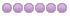# How can I make the axis movement immediate in the remapping code?

26 Jan 2022 03:27 #233144 by chen1234
I did an io signal detection in the remapping code, it can control the Z axis movement after reading a high level signal, I use the (self.execute("G0 Z %d"%b)) function to Control the Z axis, b is my parameter, but I found in the test that self.execute will not be executed in the loop, but will be executed after the loop ends, which cannot achieve my needs. I would like to know how to control the axis to make it execute immediately? Please give me some advice.
Thanks, here is my code:
```def m203(self,**words):
#yield INTERP_EXECUTE_FINISH
a = (words['s'])
b = a
c = 0
while 1：
x = hal.get_value('lcec.0.5.RXPDO-4')
if x == 1:
y = hal.get_value('lcec.0.5.RXPDO-5')
if y == 1:
while 1:
y = hal.get_value('lcec.0.5.RXPDO-5')
if y == 0:
c += 1
print("c = ", c)
if b < 44 and b >-44:
self.execute("G0 Z %d"%b)
b += a
break
elif y == 0:
time.sleep(0)
elif x == 0 or hal.get_value('lcec.0.5.TXPDO-2') == 1:
break ```

• cmorley
• Offline
• Moderator
•26 Jan 2022 06:33 #233151 by cmorley
try:
yield INTERP_EXECUTE_FINISH

after execute statement
The following user(s) said Thank You: chen1234

27 Jan 2022 02:03 - 27 Jan 2022 02:09 #233249 by chen1234
I solved the problem, I found that (self.execute("G0 Z %d"%b)) can't go to execute the Z axis movement immediately.
I solved the Z axis control delay using ( External Axis Offsets ) and it got my Z axis feeds working. I thought I should share the solution, below is my code and configuration.
remap.py:
```def m203(self,**words):
yield INTERP_EXECUTE_FINISH
a = (words['s'])
b = a
while 1 :
y = hal.get_value('lcec.0.5.RXPDO-4')
if y == 1:
x = hal.get_value('lcec.0.5.RXPDO-5')
if x == True:
while 1:
x = hal.get_value('lcec.0.5.RXPDO-5')
if x == False:
hal.set_p("axis.z.eoffset-counts","%d"%b)
print("b=",b)
b += a
break
elif x == False:
time.sleep(0)
elif y == 0 or shal.get_value('lcec.0.5.TXPDO-2') == 1:
time.sleep(0)
break
hal.set_p("axis.z.eoffset-counts","0")
```
myaxis.ini:
```[AXIS_Z]
OFFSET_AV_RATIO  =   0.2
```
myaxis.hal:
```setp axis.z.eoffset-enable 1
setp axis.z.eoffset-scale 1
```

Last edit: 27 Jan 2022 02:09 by chen1234.